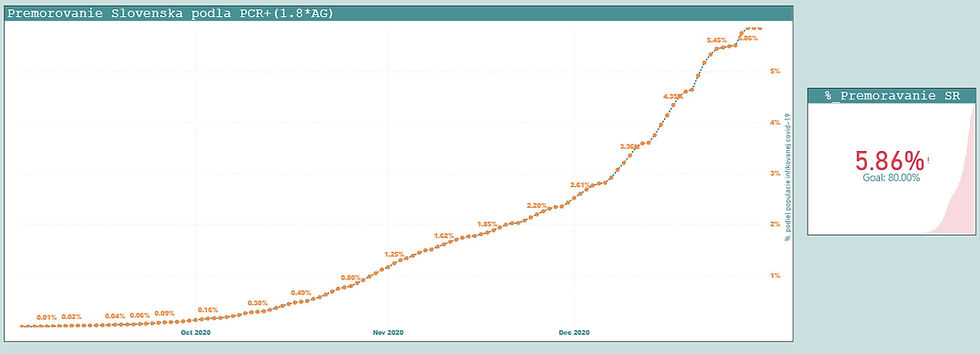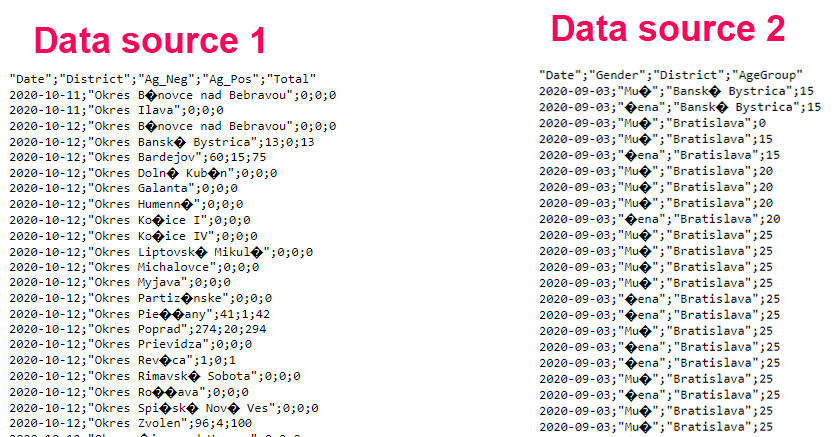top of page
Search
• Marek Vavrovic

# Covid-19, Power Bi DAX measures

Dataset source: https://www.worldometers.info/coronavirus/

Data on the server: I have been using couple of tables, this is the main one.I have created a view to display the values for the last update and the previous day (new cases – previous day new cases) but in the most cases I used DAX to create the measures in the Power BI.Recovery index measure:1: Recoveries Total =

CALCULATE (

SUM ( DimCovid[Recoveries] ),

FILTER ( DimCovid, DimCovid[Date] = MAX ( DimCovid[Date] ))

)

2: Cases Total =

CALCULATE (

SUM ( DimCovid[CasesTotal] ),

FILTER ( DimCovid, DimCovid[Date] = MAX ( DimCovid[Date] ))

)

3: RecoveryRate =

DIVIDE ( [Recoveries Total], [Cases Total], 0)

Let’s see how these measures got created.7-day median/100K

1:

Population = CALCULATE ( SUM ( WorldometersOnline[Population] ) )

2:

PopRate = [Population]/100000

3:

7-day median/100K =

VARmed =

CONVERT (

CALCULATE (

MEDIAN ( VwDimCovid[NewCases] ),

DATESINPERIOD ( VwDimCovid[Date], LASTDATE ( VwDimCovid[Date] ), -7, DAY)

),

INTEGER

)

RETURN

DIVIDE (med, [PopRate], 0)

Daily limit/100K

Safe case threshold according the EU regulation is <25/14 days. Per 1 day is 25/14=1.786Limit =

VAR lim =

DIVIDE ( [7-day median/100K], 1.786, 0)

RETURN

IF (

lim > 1,

FORMAT (lim, "0.00") & "x over limit",

IF (

lim = 1,

FORMAT (lim, "0.00") & "x =limit",

FORMAT (lim, "0.00") & "x under limit"

)

)

RN- reproductive number

New case over previous cases during some time period (7 days)

1: New Cases Last 7 Days =

CALCULATE (

SUM ( 'VWDimCovid'[NewCases] ),

DATESINPERIOD ( 'VWDimCovid'[Date], MAX ( vWDimCovid[date] ), -7, DAY )

)

2:

New Cases 8th-14th Day =

CALCULATE (

SUM ( 'VWDimCovid'[NewCases] ),

DATESINPERIOD ( 'VWDimCovid'[Date], LASTDATE ( vWDimCovid[date] ) - 7, -7, DAY)

)

3:

RN = DIVIDE([New Cases Last 7 Days],[New Cases 8th-14th Day],0)

Progress to zero

Corresponds to the percentage decline from a previously recorded peak level. The metric ranges from 0% (representing a geography that has not yet peaked) to 100% (representing a geography wherein 0 cases have been recorded for at least seven days)

1:

World7-day/avg Newcase =

CALCULATE (

AVERAGE ( vWDimCovid[NewCases] ),

DATESINPERIOD ( vWDimCovid[date], LASTDATE ( vWDimCovid[date] ), -7, DAY)

)

2:

AverageIncedenceMAXX =

MAXX (

SUMMARIZE (

vWDimCovid,

vWDimCovid[date],

"XNC", AVERAGE ( vWDimCovid[NewCases] )

),

[World7-day/avg Newcase]

)

3:

Progress to zero =

1 - ( DIVIDE ( [World7-day/avg Newcase], [AverageIncedenceMAXX], 0 ) )

progress to zero in the tooltipI was a little bit lazy to figure out average for my dataset, so I did it old statistic way…

1: InfectionIncidence =

VARday1 =

CALCULATE (

SUM ( VWDimCovid[NewCases] ),

FILTER ( vWDimCovid, VWDimCovid[date] = MAX ( VWDimCovid[date] ))

)

VARday2 =

CALCULATE (

SUM ( VWDimCovid[NewCases] ),

FILTER ( vWDimCovid, VWDimCovid[date] = MAX ( VWDimCovid[date] ) - 1)

)

VARday3 =

CALCULATE (

SUM ( VWDimCovid[NewCases] ),

FILTER ( vWDimCovid, VWDimCovid[date] = MAX ( VWDimCovid[date] ) - 2)

)

VARday4 =

CALCULATE (

SUM ( VWDimCovid[NewCases] ),

FILTER ( vWDimCovid, VWDimCovid[date] = MAX ( VWDimCovid[date] ) - 3)

)

VARday5 =

CALCULATE (

SUM ( VWDimCovid[NewCases] ),

FILTER ( vWDimCovid, VWDimCovid[date] = MAX ( VWDimCovid[date] ) - 4)

)

VARday6 =

CALCULATE (

SUM ( VWDimCovid[NewCases] ),

FILTER ( vWDimCovid, VWDimCovid[date] = MAX ( VWDimCovid[date] ) - 5)

)

VARday7 =

CALCULATE (

SUM ( VWDimCovid[NewCases] ),

FILTER ( vWDimCovid, VWDimCovid[date] = MAX ( VWDimCovid[date] ) - 6)

)

RETURN

(day1 + day2 + day3 + day4 + day5 + day6 + day7) / 7

{86400 = number of seconds in 1 day}

2:

String Duration Incedence Trend in Days Hours Minutes and Seconds =

VARvSeconds =

INT (DIVIDE (86400, [InfectionIncidence] ))

VARvMinutes =

INT (vSeconds / 60)

VARvRemainingSeconds =

MOD (vSeconds, 60)

VARvHours =

INT (vMinutes / 60)

VARvRemainingMinutes =

MOD (vMinutes, 60)

VARvDays =

INT (vHours / 24)

VARvRemainingHours =

MOD (vHours, 24)

RETURN

vDays & " Days , " & vRemainingHours & " Hrs : " & vRemainingMinutes & " Min : " & vRemainingSeconds & " Sec"

measure in the tooltipRegion / District: New cases in the last 14 days/100K

If no district is filtered the chart displays data for the region, if district is filtered the chart will show the data for a specific district.1:

2-Week/NewCases =

CALCULATE (

SUM ( DistrictGeoData[NewCases] ),

DATESINPERIOD ( DistrictGeoData[Date], MAX ( DistrictGeoData[Date] ), -14, DAY)

)

2:

NI14-day Incid/100K =

IF (

HASONEVALUE ( DistrictGeoData[District] ),

CALCULATE (DIVIDE ( [2-Week/NewCases], DistrictGeoData[DistrictPopRate] )),

CALCULATE (

DIVIDE ( [2-Week/NewCases], DistrictGeoData[RegionPopRate] ),

DistrictGeoData[Region] = "Nitriansky"

)

)

Change (KPI in the red frame) Indicates the change from the previous day.

You need a dataset with consecutive dates otherwise this code won't be working correctly.1:

Daily Confirmed =

CALCULATE (

FILTER (

)

)

2:

Daily Confirmed day before =

CALCULATE (

FILTER (

= MAX ( SlovakiaDetails[Date] ) - 1

)

)

3:

Daily confirmed change =

[Daily Confirmed] - [Daily Confirmed day before]

4:

DailyConfComment =

VAR Down =

UNICHAR (10134)

VAR Up =

UNICHAR (119108)

VAR Side =

UNICHAR (129094)

RETURN

IF (

[Daily confirmed change] > 0,

"(" & FORMAT ( [Daily confirmed change], "+#,##0") & ")",

IF (

[Daily confirmed change] < 0,

"(" & FORMAT ( [Daily confirmed change], "#,##0") & ")",

Side & " "

& FORMAT ( [Daily confirmed change], "#,##0")

)

)

Slovakia herd immunityI used 2 open sources data from GitHub (.csv) to create this visual.I took dates from the calendar tablecalculating daily new cases from PCR and AG table

1:

PCRDennePrirastky =

CALCULATE (

SUM ( PositiveTests_District[DennychPrirastkov] ),

FILTER ( 'Calendar', 'Calendar'[Date] = MAX ( PositiveTests_District[Datum] ))

)

2:

AG = CALCULATE(SUM(AgTests_District[Ag_Pos]))

I attached the measures to the tableCalculating running totals for PCR and AG test results. I created a new column for AG tests (1.8 * AG) because AG tests can only detect 50-55% of what PCR tests would detect in a similar circumstances. Don't worry about this statement.

3:

Ag_spolu =

CALCULATE (

SUM ( [50%AGplus] ),

FILTER ( Promorovanost, EARLIER ( Promorovanost[Date] ) >= Promorovanost[Date] )

)

4:

PCR_spolu =

CALCULATE (

SUM ( [PCR] ),

FILTER ( Promorovanost, EARLIER ( Promorovanost[Date] ) >= Promorovanost[Date] )

)

5: (sum of values from PCR and AG running totals )

AG_PCR_Spolu = [AG_spolu]+[PCR_spolu]

6: (Calculating the herd imunity)

%_Premorovanost =

DIVIDE ( [AG_PCR_Spolu], [SR_population], 0)

Newly created table for the visual